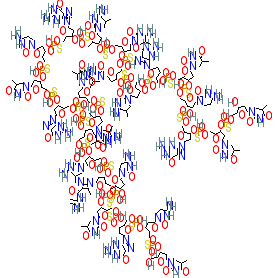#### GtoPdb Ligand ID: 9843

Synonyms: CpG 7909 | CpG7909 | PF 3512676 | PF-3512676 | ProMune | VaxImmune
Compound class: Synthetic organic
Comment: Agatolimod is synthetic CpG oligodeoxynucleotide that acts as a TLR9 agonist . We show the parent molecule, whereas the USAN specifies agatolimod sodium (PubChem CID 56841789), and the synonyms we list may also refer to the sodium congener. Its nucleotide sequence is d(P-thio)(T-C-G-T-C-G-T-T-T-T-G-T-C-G-T-T-T-T-G-T-C-G-T-T).
2D StructurePhysico-chemical PropertiesHydrogen bond acceptors 165 Hydrogen bond donors 55 Rotatable bonds 143 Topological polar surface area 3684.42 Molecular weight 7701.8 XLogP 18.21 No. Lipinski's rules broken 4
SMILES / InChI / InChIKeyCanonical SMILES OCC1OC(CC1OP(=S)(OCC1OC(CC1OP(=S)(OCC1OC(CC1OP(=S)(OCC1OC(CC1OP(=S)(OCC1OC(CC1OP(=S)(OCC1OC(CC1OP(=S)(OCC1OC(CC1OP(=S)(OCC1OC(CC1OP(=S)(OCC1OC(CC1OP(=S)(OCC1OC(CC1OP(=S)(OCC1OC(CC1OP(=S)(OCC1OC(CC1OP(=S)(OCC1OC(CC1OP(=S)(OCC1OC(CC1OP(=S)(OCC1OC(CC1OP(=S)(OCC1OC(CC1OP(=S)(OCC1OC(CC1OP(=S)(OCC1OC(CC1OP(=S)(OCC1OC(CC1OP(=S)(OCC1OC(CC1OP(=S)(OCC1OC(CC1OP(=S)(OCC1OC(CC1OP(=S)(OCC1OC(CC1OP(=S)(OCC1OC(CC1O)n1cc(C)c(=O)[nH]c1=O)O)n1cc(C)c(=O)[nH]c1=O)O)n1cnc2c1nc(N)[nH]c2=O)O)n1ccc(nc1=O)N)O)n1cc(C)c(=O)[nH]c1=O)O)n1cnc2c1nc(N)[nH]c2=O)O)n1cc(C)c(nc1=O)N)O)n1cc(C)c(nc1=O)N)O)n1cc(C)c(nc1=O)N)O)n1cc(C)c(nc1=O)N)O)n1cnc2c1nc(N)[nH]c2=O)O)n1ccc(nc1=O)N)O)n1cc(C)c(=O)[nH]c1=O)O)n1cnc2c1nc(N)[nH]c2=O)O)n1cc(C)c(=O)[nH]c1=O)O)n1cc(C)c(=O)[nH]c1=O)O)n1cc(C)c(nc1=O)N)O)n1cc(C)c(nc1=O)N)O)n1cnc(c1C)C(=O)NC(=C)N)O)n1ccc(nc1=O)N)O)n1cc(C)c(=O)[nH]c1=O)O)n1cnc2c1nc(N)[nH]c2=O)O)n1ccc(nc1=O)N)O)n1cc(C)c(=O)[nH]c1=O Isomeric SMILES OCC1OC(CC1OP(=S)(OCC1OC(CC1OP(=S)(OCC1OC(CC1OP(=S)(OCC1OC(CC1OP(=S)(OCC1OC(CC1OP(=S)(OCC1OC(CC1OP(=S)(OCC1OC(CC1OP(=S)(OCC1OC(CC1OP(=S)(OCC1OC(CC1OP(=S)(OCC1OC(CC1OP(=S)(OCC1OC(CC1OP(=S)(OCC1OC(CC1OP(=S)(OCC1OC(CC1OP(=S)(OCC1OC(CC1OP(=S)(OCC1OC(CC1OP(=S)(OCC1OC(CC1OP(=S)(OCC1OC(CC1OP(=S)(OCC1OC(CC1OP(=S)(OCC1OC(CC1OP(=S)(OCC1OC(CC1OP(=S)(OCC1OC(CC1OP(=S)(OCC1OC(CC1OP(=S)(OCC1OC(CC1OP(=S)(OCC1OC(CC1O)n1cc(C)c(=O)[nH]c1=O)O)n1cc(C)c(=O)[nH]c1=O)O)n1cnc2c1nc(N)[nH]c2=O)O)n1ccc(nc1=O)N)O)n1cc(C)c(=O)[nH]c1=O)O)n1cnc2c1nc(N)[nH]c2=O)O)n1cc(C)c(nc1=O)N)O)n1cc(C)c(nc1=O)N)O)n1cc(C)c(nc1=O)N)O)n1cc(C)c(nc1=O)N)O)n1cnc2c1nc(N)[nH]c2=O)O)n1ccc(nc1=O)N)O)n1cc(C)c(=O)[nH]c1=O)O)n1cnc2c1nc(N)[nH]c2=O)O)n1cc(C)c(=O)[nH]c1=O)O)n1cc(C)c(=O)[nH]c1=O)O)n1cc(C)c(nc1=O)N)O)n1cc(C)c(nc1=O)N)O)n1cnc(c1C)C(=O)NC(=C)N)O)n1ccc(nc1=O)N)O)n1cc(C)c(=O)[nH]c1=O)O)n1cnc2c1nc(N)[nH]c2=O)O)n1ccc(nc1=O)N)O)n1cc(C)c(=O)[nH]c1=O InChI Key GUVMFDICMFQHSZ-UHFFFAOYSA-N
 No information available.
 Summary of Clinical UseAgatolimod was investigated as an immuno-oncology therapeutic [2-3] and although manyPhase 2 clinical trials have been completed, development seems to have ceased. All trials in lung cancer were terminated by the developer in 2007, due to lack of significant efficacy in interim anaylses.